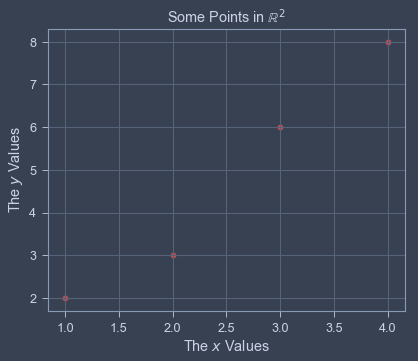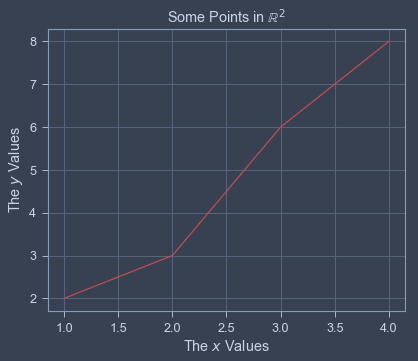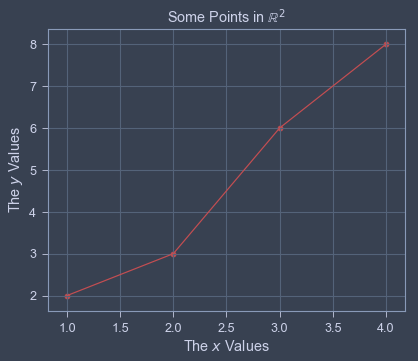Lecture 3:¶

• This lecture will cover the basics of arithmetic and basic datatypes in python
• Lists
• Ploting

Lists and Arrays¶

• Up until now, we have worked primarily with elementary data types
• A data type in this context is python's classification for what kind of object something is
• You can always check the type of an object with the conveniently named type function
• For example, type("hello") will give you str and type(2) will give you int
• Today we will explore a more complex datatype known as a list
• Lists can be used to construct datatypes that are lists of other datatypes.
• In fact, theses lists can mix datatypes
• Lets look at some examples
In :
example_list = [1,2,3,4,5]
print(example_list)
print(type(example_list))

[1, 2, 3, 4, 5]
<class 'list'>


Indexing¶

• Now suppose we wanted to access a specific element of the example list above. We would do this be referencing the elements index
• Note that Python starts counting at zero! IE, the FIRST index in the list is 0.
• This means the last index of the list is the length of the list minus 1.
• If you want to get the length of the list, you can use the len function
In :
print("The length of the list is ", len(example_list))
print('The element at index 0 is', example_list)
print('The element at index 1 is', example_list)
print('The element at index 2 is', example_list)
print('The element at index 3 is', example_list)

The length of the list is  5
The element at index 0 is 1
The element at index 1 is 2
The element at index 2 is 3
The element at index 3 is 4


Reverse Indexing¶

• Suppose we wanted to start indexing elements in an array starting from the right as opposed to the left.
• This can be accomplished by starting at -1 instead of zero
• -1 corresponds to the last element, -2 corresponds to the second to last and so on and so forth
• Note that in this case the the most negative (smallest) index value you can have is the 0 minus the length of the list
In :
print('The element at index -1 is ', example_list[-1] , 'which is the same as the element at index 3')
print('The element at index -2 is ', example_list[-2] , 'which is the same as the element at index 2')
print('The element at index -3 is ', example_list[-3] , 'which is the same as the element at index 1')
print('The element at index -4 is ', example_list[-4] , 'which is the same as the element at index 0')

The element at index -1 is  5 which is the same as the element at index 3
The element at index -2 is  4 which is the same as the element at index 2
The element at index -3 is  3 which is the same as the element at index 1
The element at index -4 is  2 which is the same as the element at index 0


Introduction to Plotting in Python¶

• There are many tools we can use to create graphs with, but for now we will stick to a package called matplotlib
• Matplotlib, in its simplest form, takes as input 2 lists of numbers.
• The first list is the $x$-coordinate of all the points you wish to plot
• The second list is the $y$-coordinate of all the points you with to plot
• For now, we focus on two main plotting functions: plt.scatter and plt.plot
• Note the dot syntax. plt is a module and the functions contained in it are scatter and plot.
• Lets take a look at the plotting syntax

Plotting Points¶

• Use the scatter(...) function to plot points from a list of x values and the associated y values
• Instead of passing $$(x_1,y_1) ,\ldots, (x_n,y_n)$$ you pass two separate lists or arrays $$[x_1,\ldots, x_n], [y_1,\ldots , y_n]$$
In :
# This importd the ploting software
# The full module name is matplotlib.pyplot but because
# we are lazy, we give it an alias plt
# that way, we don't need to type matplotlib.pyplot every time
import matplotlib.pyplot as plt

In :
# To plot the points (1,2), (2,3), (3,6), (4,8) we would list the x values and the corresponding y values:

# In python3, you can unpack assignments.
# IE, a,b,c,d = e,f,g,h  will assign a=e, b=f, c=g, d=h
xvals, yvals = [1,2,3,4], [2,3,6,8]
plt.scatter(x= xvals, y= yvals, c='r')
# we can add labels for the title, x-axis and y-axis
plt.title('Some Points in $\mathbb{R}^2$')
plt.xlabel("The $x$ Values")
plt.ylabel("The $y$ Values")
plt.show()In :
# To plot the points (1,2), (2,3), (3,6), (4,8) we would list the x values and the corresponding y values:

# In python3, you can unpack assignments.
# IE, a,b,c,d = e,f,g,h  will assign a=e, b=f, c=g, d=h
xvals, yvals = [1,2,3,4], [2,3,6,8]
plt.plot(xvals,yvals, c='r')
# we can add labels for the title, x-axis and y-axis
plt.title('Some Points in $\mathbb{R}^2$')
plt.xlabel("The $x$ Values")
plt.ylabel("The $y$ Values")
plt.show()In :
plt.plot(xvals,yvals, c='r')
plt.scatter(x= xvals, y= yvals, c='r')
# we can add labels for the title, x-axis and y-axis
plt.title('Some Points in $\mathbb{R}^2$')
plt.xlabel("The $x$ Values")
plt.ylabel("The $y$ Values")
plt.show()In [ ]: